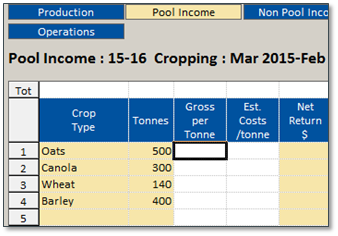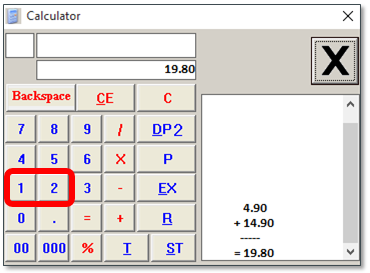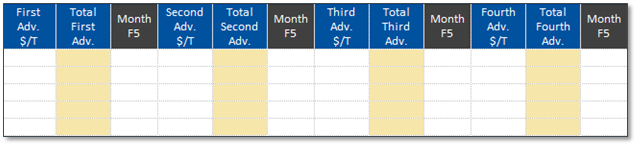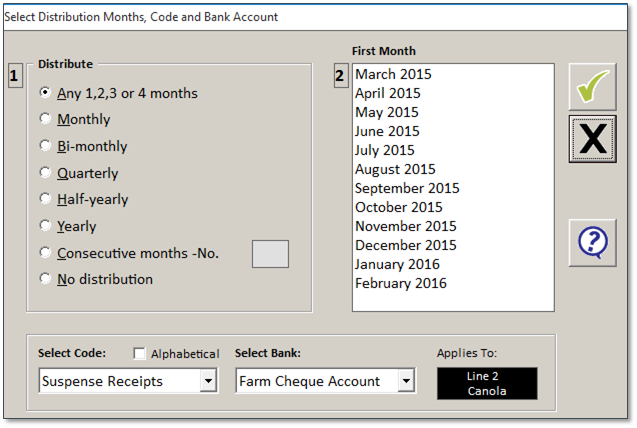Follow

# Pool Income Worksheet

The Pool Income Worksheet is used to break out the yield detail based on gross sales and any freight and storage costs. The summary information from the Crop Production Worksheet is sent into the Pool Income Worksheet. The Pool Income Worksheet gives you the option for forecasting the 4 months that the grain pool advances are paid.

Please Note: Do not complete this worksheet for tonnages that will be entered into the Grain Income Calculator. All calculations for these tonnages will be accounted for in the Grain Income Calculator.

Crop Pool Income ColumnsCrop Type Agrimaster will copy the Crop Type (crop names) from the Production Worksheet. Tonnes Agrimaster will copy the calculated Total Yield (in tonnes) from the Production Worksheet. Gross per Tonne Type the dollar amount your pool is paying per tonne for this crop.This is the net value per tonne (excluding GST) if all pool payments expected. Est Costs/Tonne Type the dollar amount for the total costs for freight, handling and storage as part of the sale of the crop to your grain pool. First Adv.\$/T Enter the expected First Advance figure, press enter. This is the expected first net advance, less all handling and selling charges. The total for the crop will be calculated. Net Return \$ Agrimaster calculates the net dollar value of the sale (Tonnes multiplied by Gross per Tonne) and subtracts the Est Costs/tonne.

Optional: Use the Calculator

Press the F3 anywhere you want a calculator to figure out a number.

For example, click into Est Costs/tonne and press F3.After calculating your total, click EX to transfer the number in.First Adv. \$/TSecond Adv. \$/TThird Adv. \$/TFourth Adv. \$/T Type the net dollar amount of the first advance in dollars per tonne. To get the net dollar amount, subtract the handling and selling charges per tonne from the sales price. Total First Adv.Total Second Adv.Total Third Adv.Total Fourth Adv. Agrimaster will calculate the total of your first advance from the pool by multiplying the Tonnes by the Adv. \$/T for this Crop Type (crop in this row). Month F5(up to 4 occurrences) Press the F5 key to select the Cashbook Code and Bank Account to which the Pool Advance will be booked against.
1. In Distribute, choose the frequency (Any 1, 2, 3 or 4 months recommended).
3. In Select Code, choose your Agrimaster Cashbook Code and the Bank Account Where the advance will be deposited.
4. Click on the green tick to ok the selections.If you are receiving grain pool advances, Agrimaster will calculate the closing payment from the grain pool.

 Later Adv. Agrimaster calculates the remaining dollars per tonne for the final advance (payment) from the grain pool. If you are tracking grain pool advances, Agrimaster adds up the Total Adv. and subtracts the advances from the Net Return \$. If you are not using advances the totals will match the calculated Net Return \$. Total Later \$ Agrimaster displays the amount of the final grain pool payment for that Crop Type. Agrimaster subtracts any money listed in any of the Total Adv. from the Net Return \$.

Click on the green tick to save the Worksheets

Please Note: Advances that do not fall into the period of this worksheet will be included in the Statement of Position. This is indicated in the Later Advances column on the far right of the worksheet.